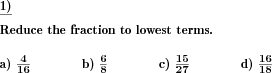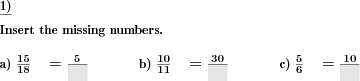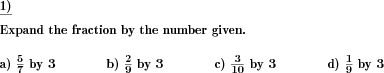Custom math worksheets at your fingertipsDetails for problem "Reduce fractions"

Quickname: 3766

Elementary School, Primary School, Junior High School, Middle School, High School.

Summary

A fraction has to be reduced to lowest terms.

ExampleDescription

Given unreduced fractions are to be reduced to lowest terms. The number to reduce the fraction by, the greatest common divisor, is not provided. It has to be determined in order to reduce the fraction properly.

The number range from which both numerator and denominator are randomly selected can be chosen.

In each problem, a single fraction is to be reduced. The number of problems and thus the number of fractions to be reduced can be specified.

Download free printable worksheets for this math problem here. The worksheet contains the problems only, the solution sheet includes the answers. Just click on the respective link.

•Worksheet 1Solution sheet with answers
•Worksheet 2Solution sheet with answers
•Worksheet 3Solution sheet with answers

If you can not see the solution sheets for download, they may be filtered out by an ad blocker that you may have installed. If this is the case, please allow ads for this page and reload the page. The solution sheets will then reappear.

• Do these sample worksheets do not really fit?
• Do you need more math worksheets, with a different level of difficulty?
• Would you like to combine different problems on a worksheet and adjust them to your needs?
• As a teacher, you can put together your own worksheets using the automatically generated math problems provided.
With a free initial credit, you can start creating your own math worksheets in a few minutes.

You can try it for free! Register here, to create custom worksheets now!

Customization options for this problem

Parameter
Possible values
Number of problems
1, 2, 3, 4, 5, 6, 7, 8, 9, 10
Number range
20, 30, 40, 50, 100, 200, 500, 1000, 10000, 100000

Similar problems

Remark
Description
Similar but with given number to reduce by
Fractions are to be reduced by a given number

dw-Math worksheet templates that do contain this problem

Name
Title
Description
Reducing and expanding fractions
Expansion and reduction of fractions, and converting fractions to like quantities.

Other types of problems that appear on worksheets with this problem:

Relevance
Name
Description
Quickname
Example
****
Missing elements in unlike fractions
In unlike fractions of the same value, numerators or denominators have to be filled in.****
Convert fractions to like fractions
Convert multiple fractions to like quantities**
Expand fractions by given number
Fractions are to be expanded by a given numberDeutsche Version dieser Aufgabe
These informational pages with samples describe math problems that can be combined on custom math worksheets with solutions for home and K-12 school use.
Deutsche Seiten
×